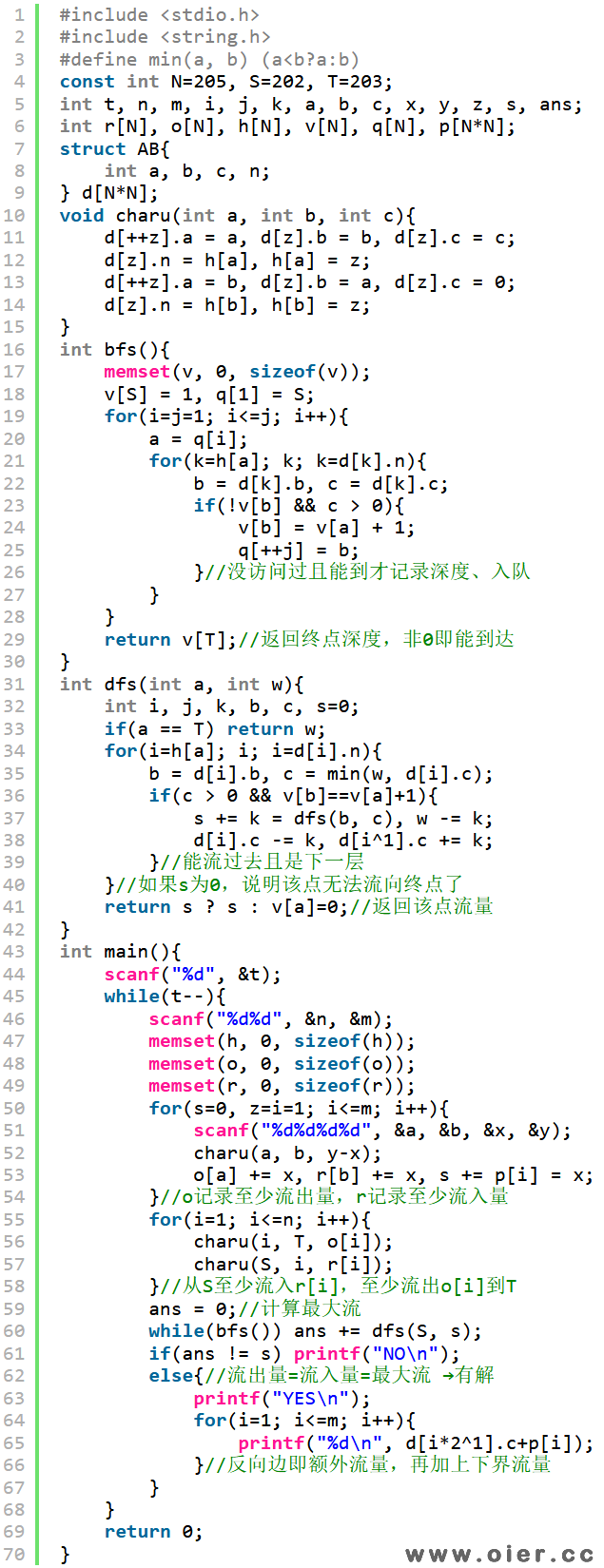ZOJ2314ReactorCooling
3369+

The terrorist group leaded by a well known international terrorist Ben Bladen is buliding a nuclear reactor to produce plutonium for the nuclear bomb they are planning to create. Being the wicked computer genius of this group, you are responsible for developing the cooling system for the reactor.

The cooling system of the reactor consists of the number of pipes that special cooling liquid flows by. Pipes are connected at special points, called nodes, each pipe has the starting node and the end point. The liquid must flow by the pipe from its start point to its end point and not in the opposite direction.

Let the nodes be numbered from 1 to N. The cooling system must be designed so that the liquid is circulating by the pipes and the amount of the liquid coming to each node (in the unit of time) is equal to the amount of liquid leaving the node. That is, if we designate the amount of liquid going by the pipe from i-th node to j-th as fij, (put fij = 0 if there is no pipe from node i to node j), for each i the following condition must hold:

fi,1+fi,2+…+fi,N = f1,i+f2,i+…+fN,i
Each pipe has some finite capacity, therefore for each i and j connected by the pipe must be fij <= cij where cij is the capacity of the pipe. To provide sufficient cooling, the amount of the liquid flowing by the pipe going from i-th to j-th nodes must be at least lij, thus it must be fij >= lij.

Given cij and lij for all pipes, find the amount fij, satisfying the conditions specified above.

This problem contains multiple test cases!

The first line of a multiple input is an integer N, then a blank line followed by N input blocks. Each input block is in the format indicated in the problem description. There is a blank line between input blocks.

The output format consists of N output blocks. There is a blank line between output blocks.

### Input

The first line of the input file contains the number N (1 <= N <= 200) – the number of nodes and and M – the number of pipes. The following M lines contain four integer number each – i, j, lij and cij each. There is at most one pipe connecting any two nodes and 0 <= lij <= cij <= 10^5 for all pipes. No pipe connects a node to itself. If there is a pipe from i-th node to j-th, there is no pipe from j-th node to i-th.

### Output

On the first line of the output file print YES if there is the way to carry out reactor cooling and NO if there is none. In the first case M integers must follow, k-th number being the amount of liquid flowing by the k-th pipe. Pipes are numbered as they are given in the input file.

### Sample Input

2
4 6
1 2 1 2
2 3 1 2
3 4 1 2
4 1 1 2
1 3 1 2
4 2 1 2
4 6
1 2 1 3
2 3 1 3
3 4 1 3
4 1 1 3
1 3 1 3
4 2 1 3

### Sample Input

NO
YES
1
2
3
2
1
1

# 解题思路

S能流到T，说明下界满足，那怎么找可行流呢？对于每一条边，除了必须要流的（下界），还有可以流的，如果反向边有流量，说明该边流出了流量——自由流，其总流量为自由流流量加上下界流量。

# 程序实现